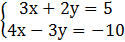### Sample Problem

Solve using the elimination method:Give your answers in simplified improper fraction form.

x=

y=

#### SolutionTo obtain the same coefficients, multiply the first equation by 4 and the second equation by 3 (to get 12x in both equations). Then subtract the two equations from one another.

4(3x+2y)=5(4)=12x+8y=20

3(4x-3y)=3(-10)=12x-9y=-30

12x+8y=20

-(12x-9y=-30)

=17y=50

y=50/17

By back substituting y=50/17 into either equation, we can solve for x.

3x+2y=5

3x=5-2(50/17)=-15/17

x=-5/17

x=-5/17 ; y=50/17